# Selina Solutions Concise Maths Class 7 Chapter 7: Unitary Method (Including Time and Work)

Selina Solutions Concise Maths Class 7 Chapter 7 Unitary Method (Including Time and Work) is prepared by teachers at BYJU’S in an interactive manner to make learning fun for the students. The solutions help students improve their problem solving abilities, which are important from the exam point of view. Students can download Selina Solutions Concise Maths Class 7 Chapter 7 Unitary Method (Including Time and Work) free PDF, from the links which are given below.

Chapter 7 mainly explains the concept of types of variations and problems on time and work. The solutions PDF is present in both chapter and exercise wise format to boost the exam preparation of students.

## Selina Solutions Concise Maths Class 7 Chapter 7: Unitary Method (Including Time and Work) Download PDF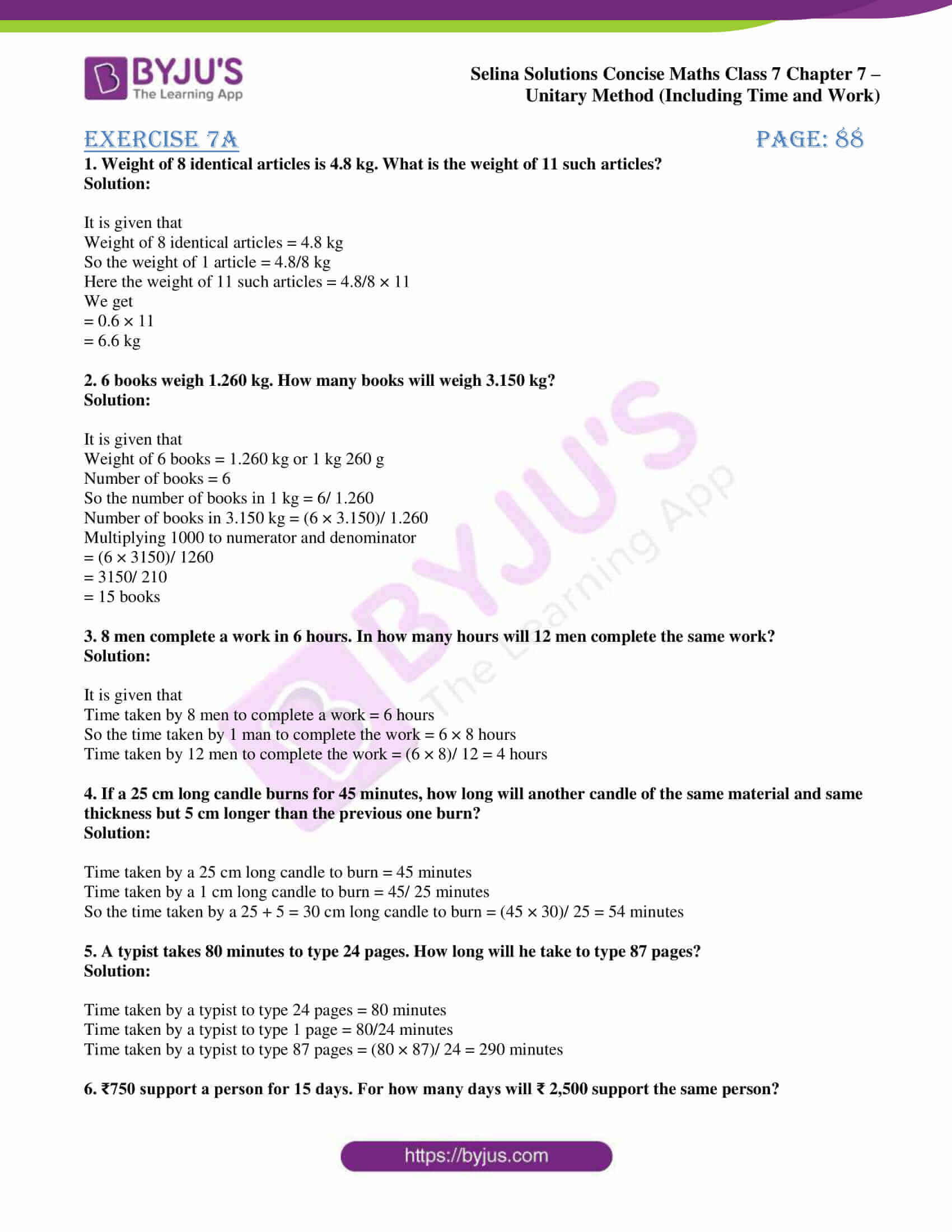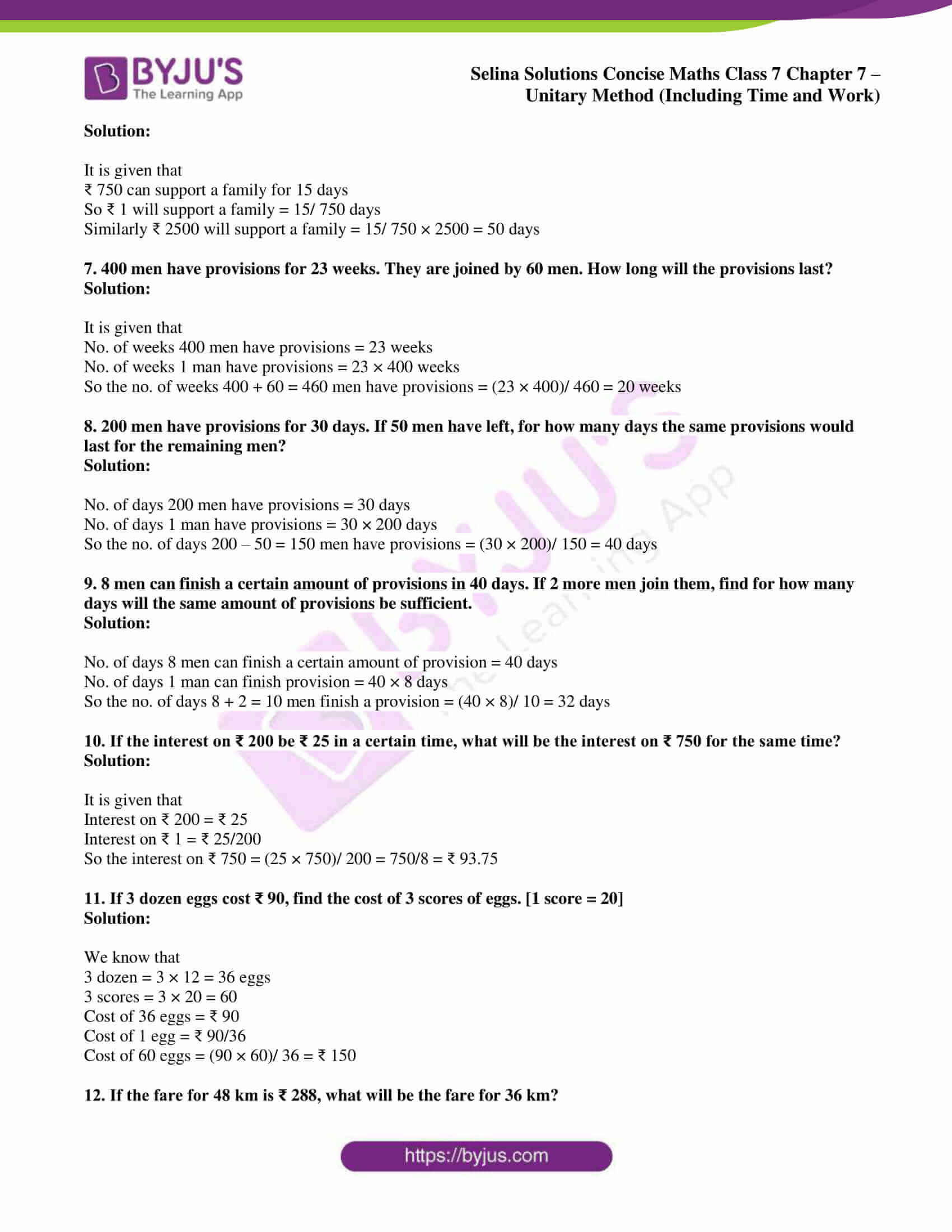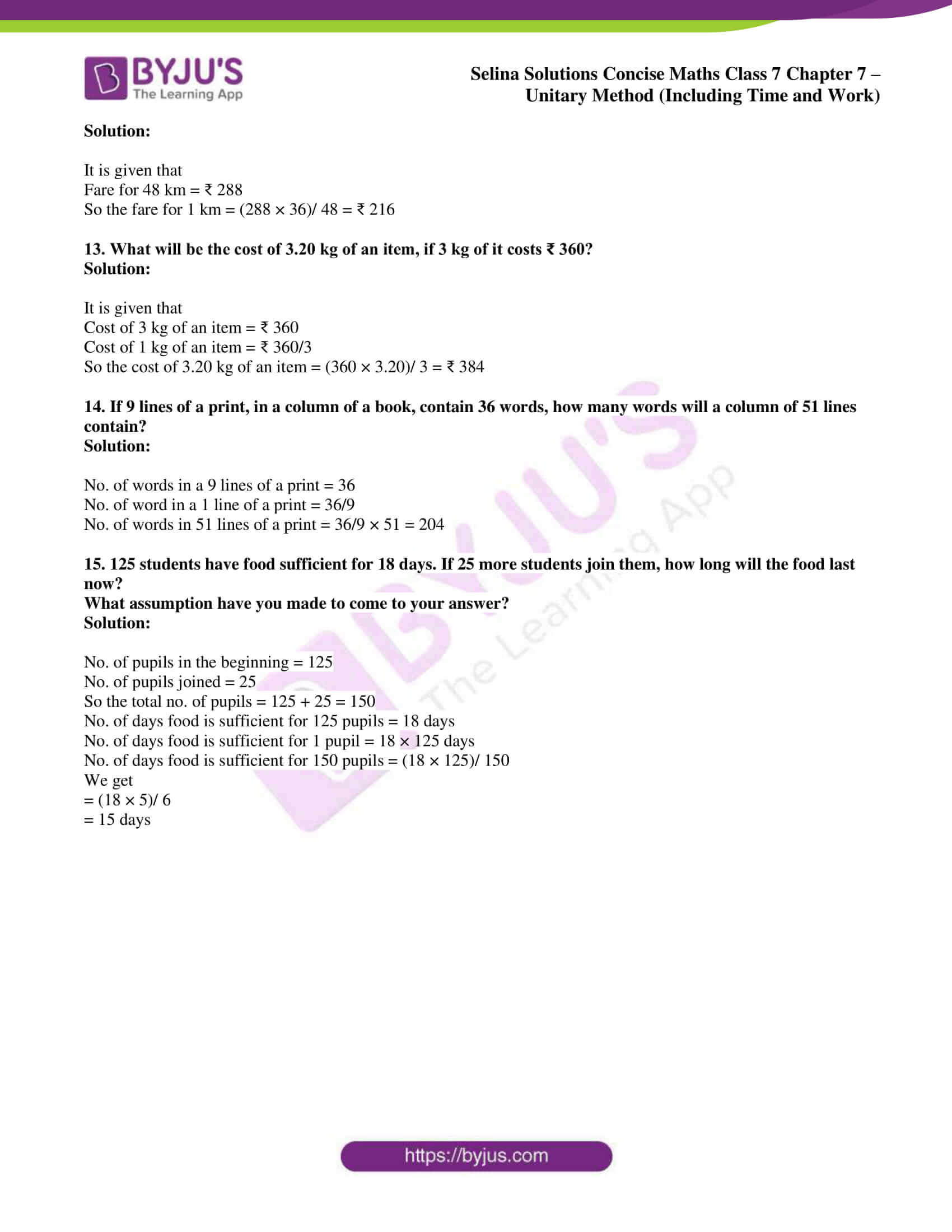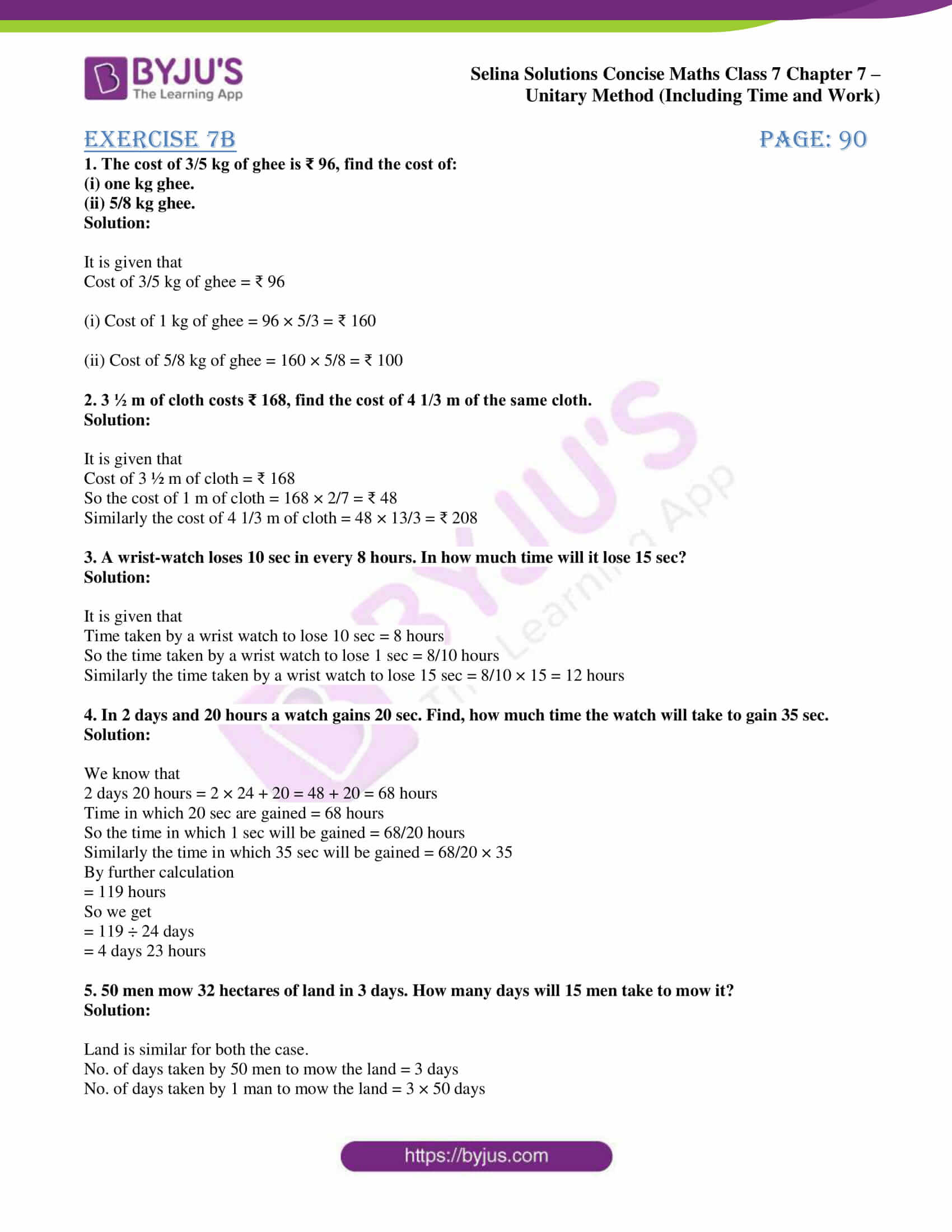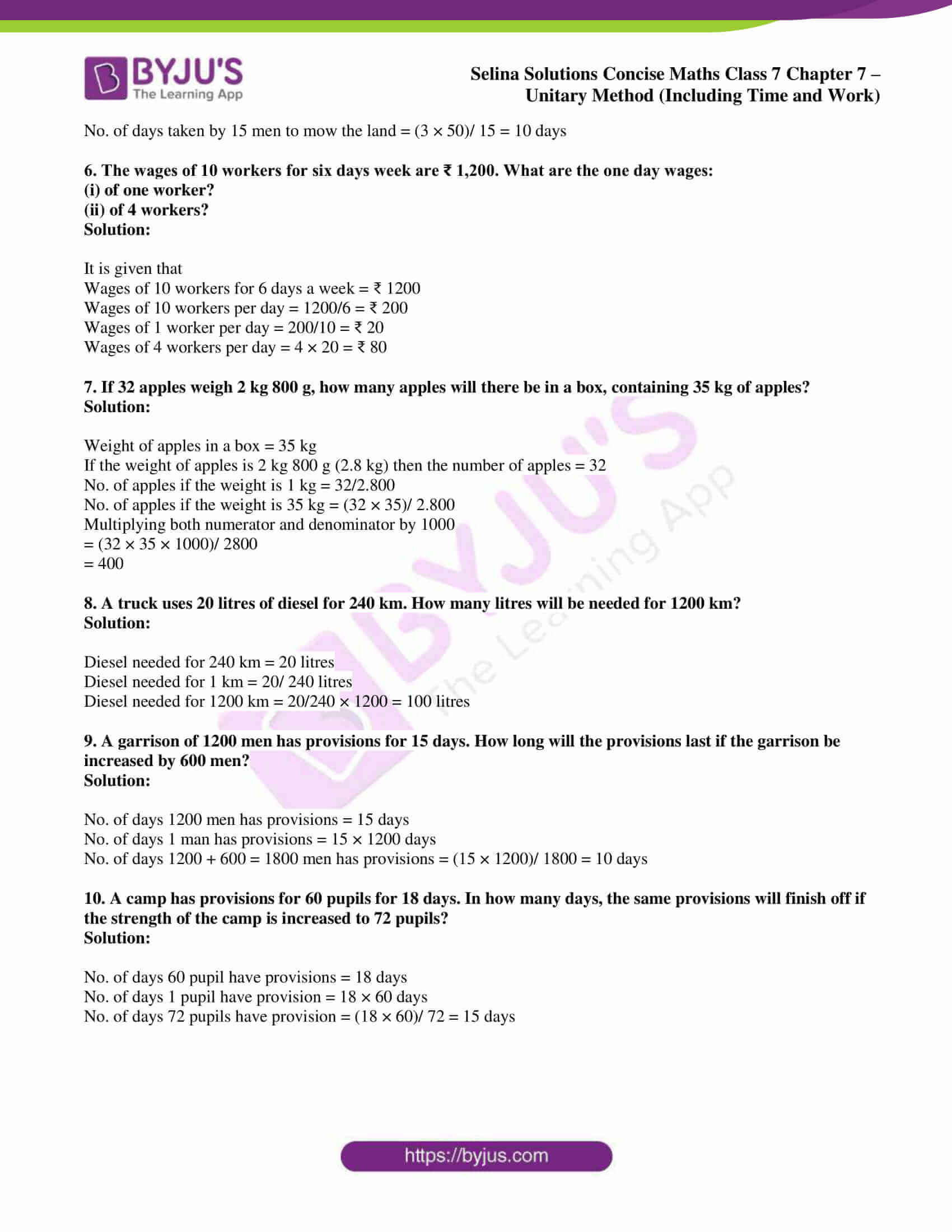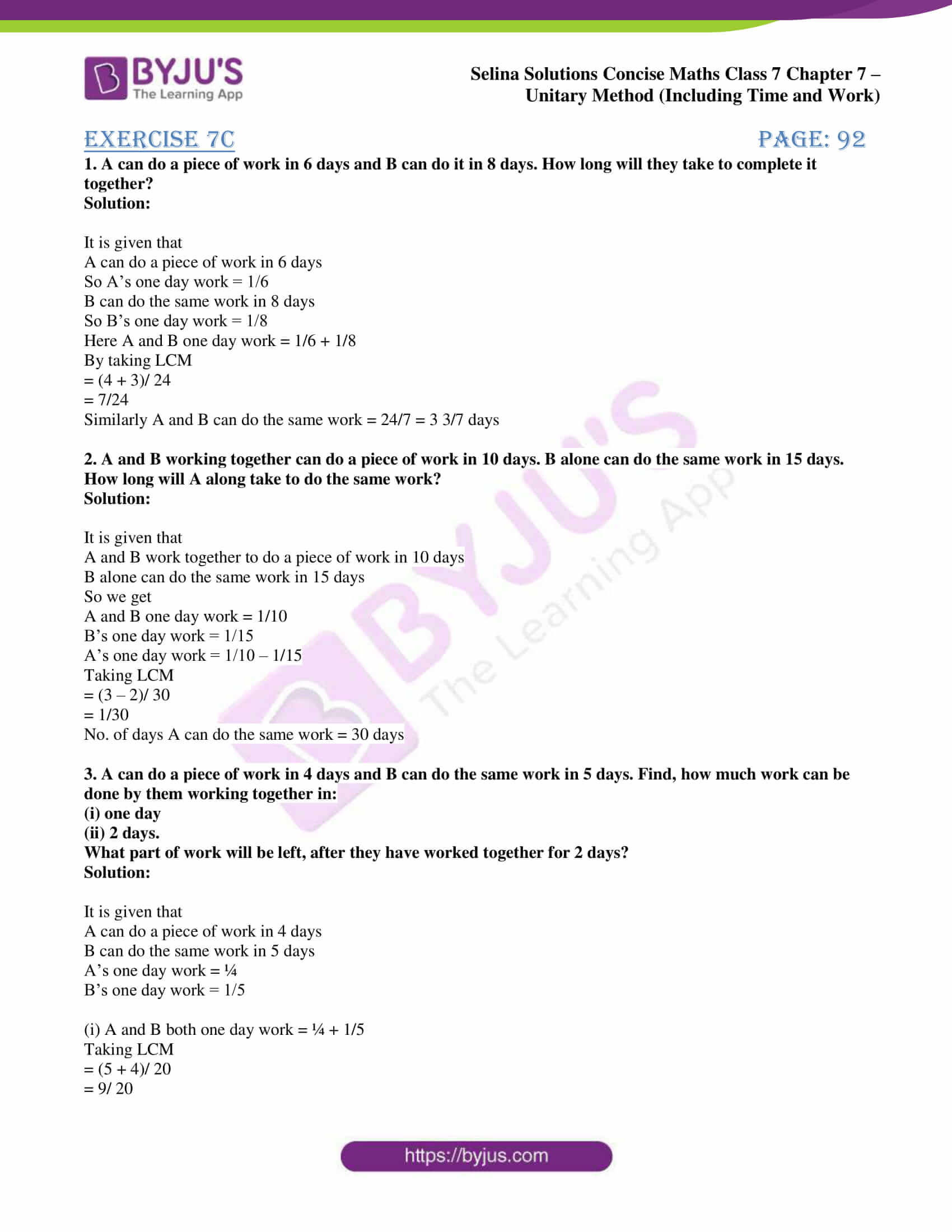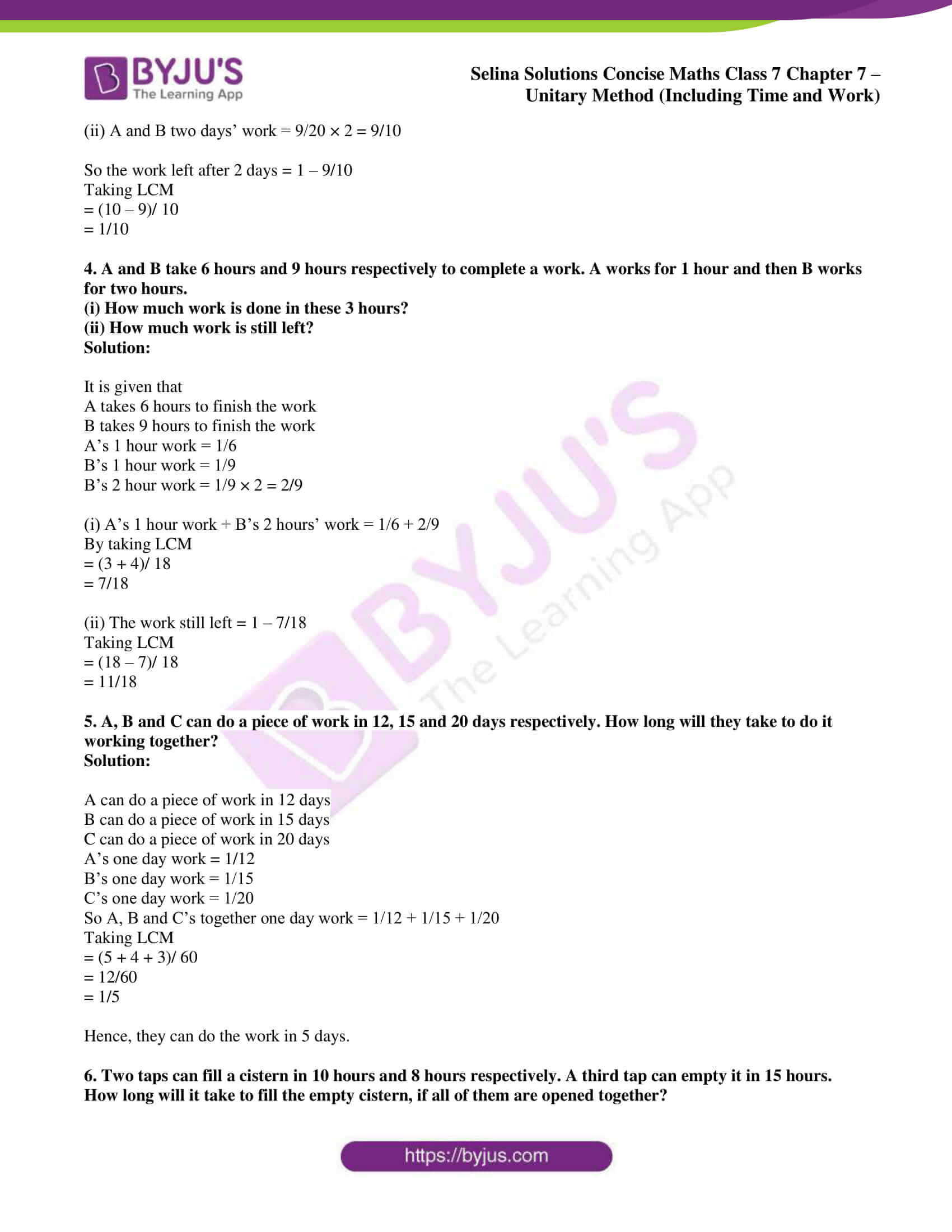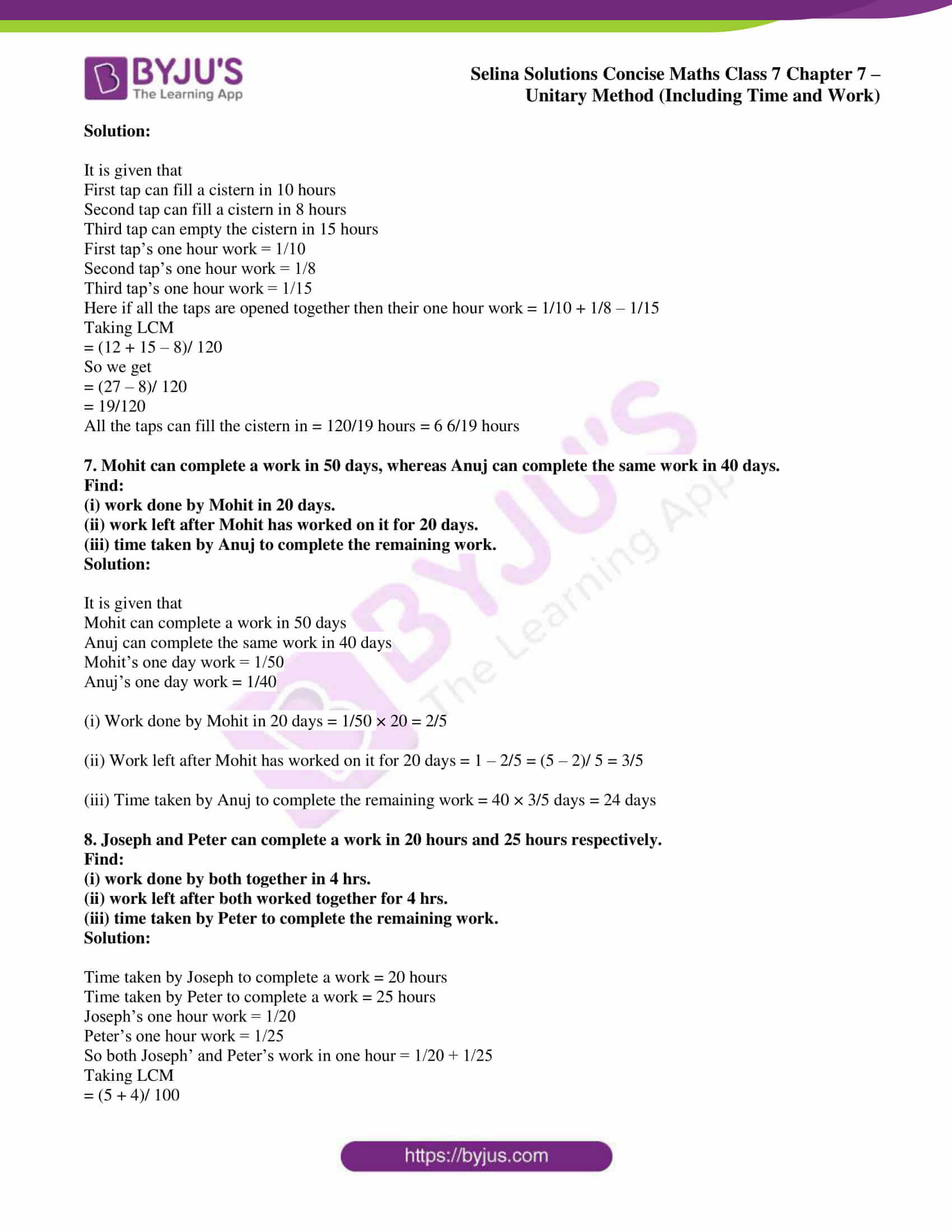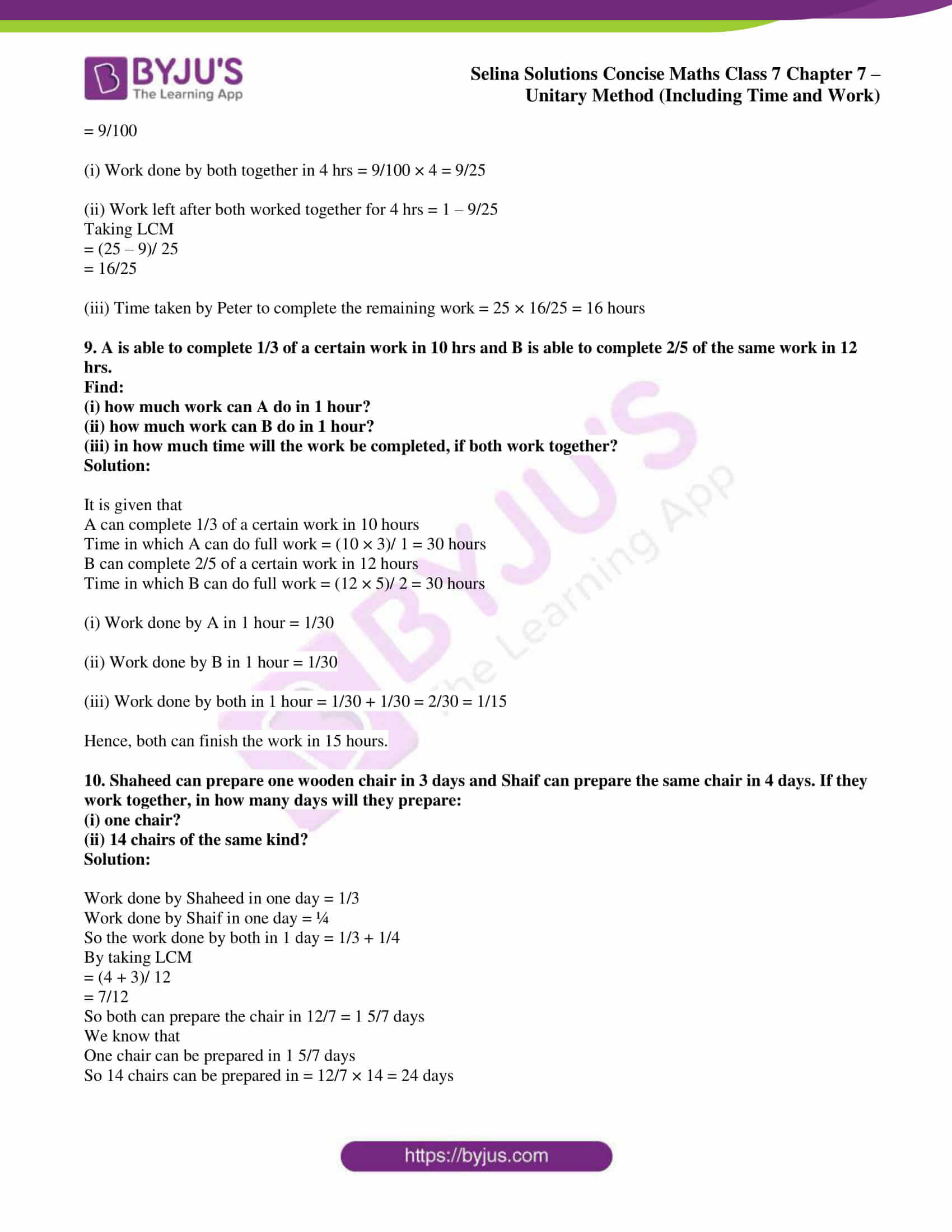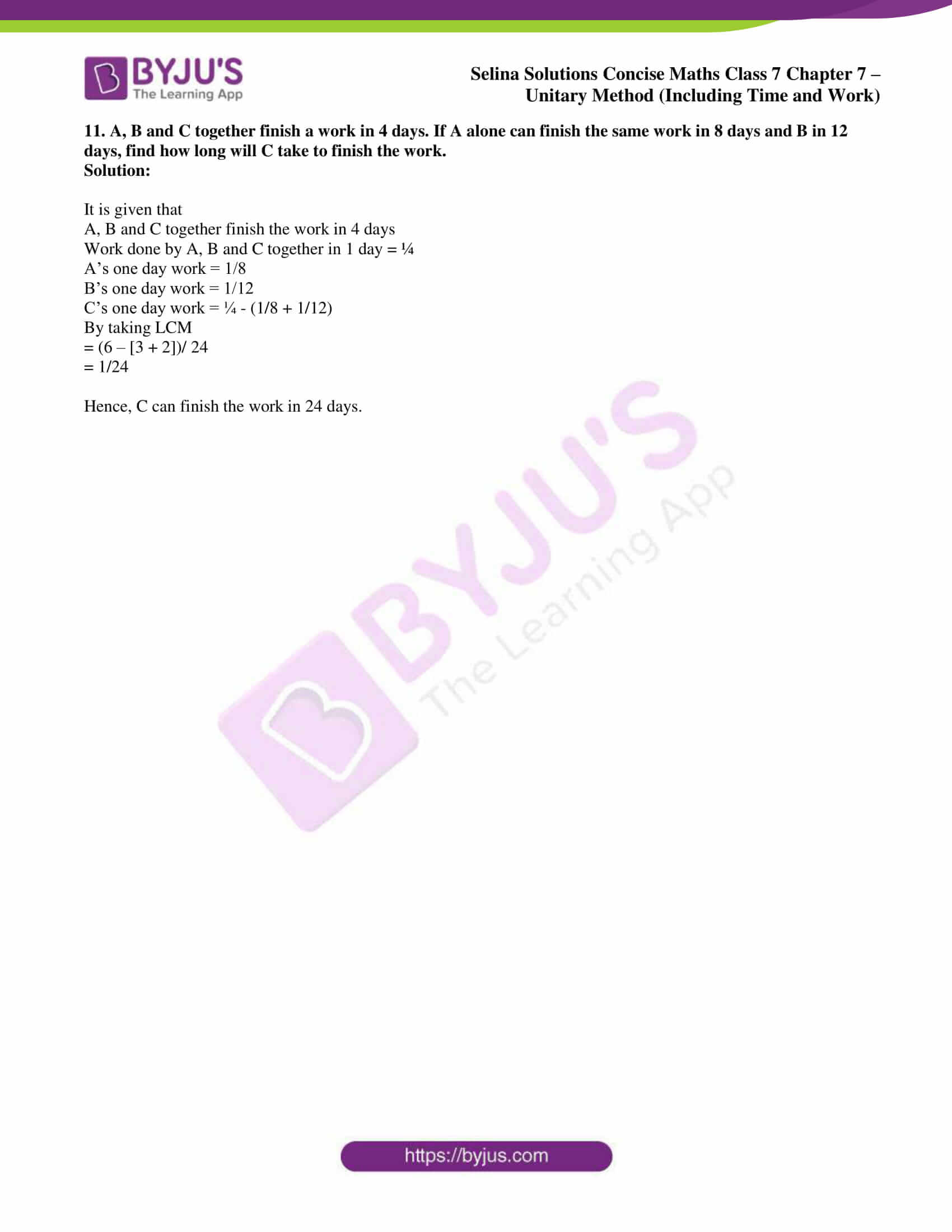## Access Selina Solutions Concise Maths Class 7 Chapter 7: Unitary Method (Including Time and Work)

#### Exercise 7A page: 88

1. Weight of 8 identical articles is 4.8 kg. What is the weight of 11 such articles?

Solution:

It is given that

Weight of 8 identical articles = 4.8 kg

So the weight of 1 article = 4.8/8 kg

Here the weight of 11 such articles = 4.8/8 × 11

We get

= 0.6 × 11

= 6.6 kg

2. 6 books weigh 1.260 kg. How many books will weigh 3.150 kg?

Solution:

It is given that

Weight of 6 books = 1.260 kg or 1 kg 260 g

Number of books = 6

So the number of books in 1 kg = 6/ 1.260

Number of books in 3.150 kg = (6 × 3.150)/ 1.260

Multiplying 1000 to numerator and denominator

= (6 × 3150)/ 1260

= 3150/ 210

= 15 books

3. 8 men complete a work in 6 hours. In how many hours will 12 men complete the same work?

Solution:

It is given that

Time taken by 8 men to complete a work = 6 hours

So the time taken by 1 man to complete the work = 6 × 8 hours

Time taken by 12 men to complete the work = (6 × 8)/ 12 = 4 hours

4. If a 25 cm long candle burns for 45 minutes, how long will another candle of the same material and same thickness but 5 cm longer than the previous one burn?

Solution:

Time taken by a 25 cm long candle to burn = 45 minutes

Time taken by a 1 cm long candle to burn = 45/ 25 minutes

So the time taken by a 25 + 5 = 30 cm long candle to burn = (45 × 30)/ 25 = 54 minutes

5. A typist takes 80 minutes to type 24 pages. How long will he take to type 87 pages?

Solution:

Time taken by a typist to type 24 pages = 80 minutes

Time taken by a typist to type 1 page = 80/24 minutes

Time taken by a typist to type 87 pages = (80 × 87)/ 24 = 290 minutes

6. ₹750 support a person for 15 days. For how many days will ₹ 2,500 support the same person?

Solution:

It is given that

₹ 750 can support a family for 15 days

So ₹ 1 will support a family = 15/ 750 days

Similarly ₹ 2500 will support a family = 15/ 750 × 2500 = 50 days

7. 400 men have provisions for 23 weeks. They are joined by 60 men. How long will the provisions last?

Solution:

It is given that

No. of weeks 400 men have provisions = 23 weeks

No. of weeks 1 man have provisions = 23 × 400 weeks

So the no. of weeks 400 + 60 = 460 men have provisions = (23 × 400)/ 460 = 20 weeks

8. 200 men have provisions for 30 days. If 50 men have left, for how many days the same provisions would last for the remaining men?

Solution:

No. of days 200 men have provisions = 30 days

No. of days 1 man have provisions = 30 × 200 days

So the no. of days 200 – 50 = 150 men have provisions = (30 × 200)/ 150 = 40 days

9. 8 men can finish a certain amount of provisions in 40 days. If 2 more men join them, find for how many days will the same amount of provisions be sufficient.

Solution:

No. of days 8 men can finish a certain amount of provision = 40 days

No. of days 1 man can finish provision = 40 × 8 days

So the no. of days 8 + 2 = 10 men finish a provision = (40 × 8)/ 10 = 32 days

10. If the interest on ₹ 200 be ₹ 25 in a certain time, what will be the interest on ₹ 750 for the same time?

Solution:

It is given that

Interest on ₹ 200 = ₹ 25

Interest on ₹ 1 = ₹ 25/200

So the interest on ₹ 750 = (25 × 750)/ 200 = 750/8 = ₹ 93.75

11. If 3 dozen eggs cost ₹ 90, find the cost of 3 scores of eggs. [1 score = 20]

Solution:

We know that

3 dozen = 3 × 12 = 36 eggs

3 scores = 3 × 20 = 60

Cost of 36 eggs = ₹ 90

Cost of 1 egg = ₹ 90/36

Cost of 60 eggs = (90 × 60)/ 36 = ₹ 150

12. If the fare for 48 km is ₹ 288, what will be the fare for 36 km?

Solution:

It is given that

Fare for 48 km = ₹ 288

So the fare for 1 km = (288 × 36)/ 48 = ₹ 216

13. What will be the cost of 3.20 kg of an item, if 3 kg of it costs ₹ 360?

Solution:

It is given that

Cost of 3 kg of an item = ₹ 360

Cost of 1 kg of an item = ₹ 360/3

So the cost of 3.20 kg of an item = (360 × 3.20)/ 3 = ₹ 384

14. If 9 lines of a print, in a column of a book, contain 36 words, how many words will a column of 51 lines contain?

Solution:

No. of words in a 9 lines of a print = 36

No. of word in a 1 line of a print = 36/9

No. of words in 51 lines of a print = 36/9 × 51 = 204

15. 125 students have food sufficient for 18 days. If 25 more students join them, how long will the food last now?

Solution:

No. of pupils in the beginning = 125

No. of pupils joined = 25

So the total no. of pupils = 125 + 25 = 150

No. of days food is sufficient for 125 pupils = 18 days

No. of days food is sufficient for 1 pupil = 18 × 125 days

No. of days food is sufficient for 150 pupils = (18 × 125)/ 150

We get

= (18 × 5)/ 6

= 15 days

#### Exercise 7B page: 90

1. The cost of 3/5 kg of ghee is ₹ 96, find the cost of:

(i) one kg ghee.

(ii) 5/8 kg ghee.

Solution:

It is given that

Cost of 3/5 kg of ghee = ₹ 96

(i) Cost of 1 kg of ghee = 96 × 5/3 = ₹ 160

(ii) Cost of 5/8 kg of ghee = 160 × 5/8 = ₹ 100

2. 3 ½ m of cloth costs ₹ 168, find the cost of 4 1/3 m of the same cloth.

Solution:

It is given that

Cost of 3 ½ m of cloth = ₹ 168

So the cost of 1 m of cloth = 168 × 2/7 = ₹ 48

Similarly the cost of 4 1/3 m of cloth = 48 × 13/3 = ₹ 208

3. A wrist-watch loses 10 sec in every 8 hours. In how much time will it lose 15 sec?

Solution:

It is given that

Time taken by a wrist watch to lose 10 sec = 8 hours

So the time taken by a wrist watch to lose 1 sec = 8/10 hours

Similarly the time taken by a wrist watch to lose 15 sec = 8/10 × 15 = 12 hours

4. In 2 days and 20 hours a watch gains 20 sec. Find, how much time the watch will take to gain 35 sec.

Solution:

We know that

2 days 20 hours = 2 × 24 + 20 = 48 + 20 = 68 hours

Time in which 20 sec are gained = 68 hours

So the time in which 1 sec will be gained = 68/20 hours

Similarly the time in which 35 sec will be gained = 68/20 × 35

By further calculation

= 119 hours

So we get

= 119 ÷ 24 days

= 4 days 23 hours

5. 50 men mow 32 hectares of land in 3 days. How many days will 15 men take to mow it?

Solution:

Land is similar for both the case.

No. of days taken by 50 men to mow the land = 3 days

No. of days taken by 1 man to mow the land = 3 × 50 days

No. of days taken by 15 men to mow the land = (3 × 50)/ 15 = 10 days

6. The wages of 10 workers for six days week are ₹ 1,200. What are the one day wages:

(i) of one worker?

(ii) of 4 workers?

Solution:

It is given that

Wages of 10 workers for 6 days a week = ₹ 1200

Wages of 10 workers per day = 1200/6 = ₹ 200

Wages of 1 worker per day = 200/10 = ₹ 20

Wages of 4 workers per day = 4 × 20 = ₹ 80

7. If 32 apples weigh 2 kg 800 g, how many apples will there be in a box, containing 35 kg of apples?

Solution:

Weight of apples in a box = 35 kg

If the weight of apples is 2 kg 800 g (2.8 kg) then the number of apples = 32

No. of apples if the weight is 1 kg = 32/2.800

No. of apples if the weight is 35 kg = (32 × 35)/ 2.800

Multiplying both numerator and denominator by 1000

= (32 × 35 × 1000)/ 2800

= 400

8. A truck uses 20 litres of diesel for 240 km. How many litres will be needed for 1200 km?

Solution:

Diesel needed for 240 km = 20 litres

Diesel needed for 1 km = 20/ 240 litres

Diesel needed for 1200 km = 20/240 × 1200 = 100 litres

9. A garrison of 1200 men has provisions for 15 days. How long will the provisions last if the garrison be increased by 600 men?

Solution:

No. of days 1200 men has provisions = 15 days

No. of days 1 man has provisions = 15 × 1200 days

No. of days 1200 + 600 = 1800 men has provisions = (15 × 1200)/ 1800 = 10 days

10. A camp has provisions for 60 pupils for 18 days. In how many days, the same provisions will finish off if the strength of the camp is increased to 72 pupils?

Solution:

No. of days 60 pupil have provisions = 18 days

No. of days 1 pupil have provision = 18 × 60 days

No. of days 72 pupils have provision = (18 × 60)/ 72 = 15 days

#### Exercise 7C page: 92

1. A can do a piece of work in 6 days and B can do it in 8 days. How long will they take to complete it together?

Solution:

It is given that

A can do a piece of work in 6 days

So A’s one day work = 1/6

B can do the same work in 8 days

So B’s one day work = 1/8

Here A and B one day work = 1/6 + 1/8

By taking LCM

= (4 + 3)/ 24

= 7/24

Similarly A and B can do the same work = 24/7 = 3 3/7 days

2. A and B working together can do a piece of work in 10 days. B alone can do the same work in 15 days. How long will A along take to do the same work?

Solution:

It is given that

A and B work together to do a piece of work in 10 days

B alone can do the same work in 15 days

So we get

A and B one day work = 1/10

B’s one day work = 1/15

A’s one day work = 1/10 – 1/15

Taking LCM

= (3 – 2)/ 30

= 1/30

No. of days A can do the same work = 30 days

3. A can do a piece of work in 4 days and B can do the same work in 5 days. Find, how much work can be done by them working together in:

(i) one day

(ii) 2 days.

What part of work will be left, after they have worked together for 2 days?

Solution:

It is given that

A can do a piece of work in 4 days

B can do the same work in 5 days

A’s one day work = ¼

B’s one day work = 1/5

(i) A and B both one day work = ¼ + 1/5

Taking LCM

= (5 + 4)/ 20

= 9/ 20

(ii) A and B two days’ work = 9/20 × 2 = 9/10

So the work left after 2 days = 1 – 9/10

Taking LCM

= (10 – 9)/ 10

= 1/10

4. A and B take 6 hours and 9 hours respectively to complete a work. A works for 1 hour and then B works for two hours.

(i) How much work is done in these 3 hours?

(ii) How much work is still left?

Solution:

It is given that

A takes 6 hours to finish the work

B takes 9 hours to finish the work

A’s 1 hour work = 1/6

B’s 1 hour work = 1/9

B’s 2 hour work = 1/9 × 2 = 2/9

(i) A’s 1 hour work + B’s 2 hours’ work = 1/6 + 2/9

By taking LCM

= (3 + 4)/ 18

= 7/18

(ii) The work still left = 1 – 7/18

Taking LCM

= (18 – 7)/ 18

= 11/18

5. A, B and C can do a piece of work in 12, 15 and 20 days respectively. How long will they take to do it working together?

Solution:

A can do a piece of work in 12 days

B can do a piece of work in 15 days

C can do a piece of work in 20 days

A’s one day work = 1/12

B’s one day work = 1/15

C’s one day work = 1/20

So A, B and C’s together one day work = 1/12 + 1/15 + 1/20

Taking LCM

= (5 + 4 + 3)/ 60

= 12/60

= 1/5

Hence, they can do the work in 5 days.

6. Two taps can fill a cistern in 10 hours and 8 hours respectively. A third tap can empty it in 15 hours. How long will it take to fill the empty cistern, if all of them are opened together?

Solution:

It is given that

First tap can fill a cistern in 10 hours

Second tap can fill a cistern in 8 hours

Third tap can empty the cistern in 15 hours

First tap’s one hour work = 1/10

Second tap’s one hour work = 1/8

Third tap’s one hour work = 1/15

Here if all the taps are opened together then their one hour work = 1/10 + 1/8 – 1/15

Taking LCM

= (12 + 15 – 8)/ 120

So we get

= (27 – 8)/ 120

= 19/120

All the taps can fill the cistern in = 120/19 hours = 6 6/19 hours

7. Mohit can complete a work in 50 days, whereas Anuj can complete the same work in 40 days.

Find:

(i) work done by Mohit in 20 days.

(ii) work left after Mohit has worked on it for 20 days.

(iii) time taken by Anuj to complete the remaining work.

Solution:

It is given that

Mohit can complete a work in 50 days

Anuj can complete the same work in 40 days

Mohit’s one day work = 1/50

Anuj’s one day work = 1/40

(i) Work done by Mohit in 20 days = 1/50 × 20 = 2/5

(ii) Work left after Mohit has worked on it for 20 days = 1 – 2/5 = (5 – 2)/ 5 = 3/5

(iii) Time taken by Anuj to complete the remaining work = 40 × 3/5 days = 24 days

8. Joseph and Peter can complete a work in 20 hours and 25 hours respectively.

Find:

(i) work done by both together in 4 hrs.

(ii) work left after both worked together for 4 hrs.

(iii) time taken by Peter to complete the remaining work.

Solution:

Time taken by Joseph to complete a work = 20 hours

Time taken by Peter to complete a work = 25 hours

Joseph’s one hour work = 1/20

Peter’s one hour work = 1/25

So both Joseph’ and Peter’s work in one hour = 1/20 + 1/25

Taking LCM

= (5 + 4)/ 100

= 9/100

(i) Work done by both together in 4 hrs = 9/100 × 4 = 9/25

(ii) Work left after both worked together for 4 hrs = 1 – 9/25

Taking LCM

= (25 – 9)/ 25

= 16/25

(iii) Time taken by Peter to complete the remaining work = 25 × 16/25 = 16 hours

9. A is able to complete 1/3 of a certain work in 10 hrs and B is able to complete 2/5 of the same work in 12 hrs.

Find:

(i) how much work can A do in 1 hour?

(ii) how much work can B do in 1 hour?

(iii) in how much time will the work be completed, if both work together?

Solution:

It is given that

A can complete 1/3 of a certain work in 10 hours

Time in which A can do full work = (10 × 3)/ 1 = 30 hours

B can complete 2/5 of a certain work in 12 hours

Time in which B can do full work = (12 × 5)/ 2 = 30 hours

(i) Work done by A in 1 hour = 1/30

(ii) Work done by B in 1 hour = 1/30

(iii) Work done by both in 1 hour = 1/30 + 1/30 = 2/30 = 1/15

Hence, both can finish the work in 15 hours.

10. Shaheed can prepare one wooden chair in 3 days and Shaif can prepare the same chair in 4 days. If they work together, in how many days will they prepare:

(i) one chair?

(ii) 14 chairs of the same kind?

Solution:

Work done by Shaheed in one day = 1/3

Work done by Shaif in one day = ¼

So the work done by both in 1 day = 1/3 + 1/4

By taking LCM

= (4 + 3)/ 12

= 7/12

So both can prepare the chair in 12/7 = 1 5/7 days

We know that

One chair can be prepared in 1 5/7 days

So 14 chairs can be prepared in = 12/7 × 14 = 24 days

11. A, B and C together finish a work in 4 days. If A alone can finish the same work in 8 days and B in 12 days, find how long will C take to finish the work.

Solution:

It is given that

A, B and C together finish the work in 4 days

Work done by A, B and C together in 1 day = ¼

A’s one day work = 1/8

B’s one day work = 1/12

C’s one day work = ¼ – (1/8 + 1/12)

By taking LCM

= (6 – [3 + 2])/ 24

= 1/24

Hence, C can finish the work in 24 days.